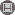دوره 10، شماره 2 - ( 7-1394 )                   جلد 10 شماره 2 صفحات 23-27 | برگشت به فهرست نسخه ها

BibTeX | RIS | EndNote | Medlars | ProCite | Reference Manager | RefWorks
Send citation to:Sheikh Hosseini A. A Generalized Singular Value Inequality for Heinz Means. IJMSI. 2015; 10 (2) :23-27
URL: http://ijmsi.ir/article-1-464-fa.html
A Generalized Singular Value Inequality for Heinz Means. مجله علوم ریاضی و انفورماتیک. 1394; 10 (2) :23-27

چکیده:

In this paper we will generalize a singular value inequality that was proved before. In particular we obtain an inequality for numerical radius as follows: begin{equation*} 2 sqrt{t (1-t)} omega(t A^{nu}B^{1-nu}+(1-t)A^{1-nu}B^{nu}) leq omega(t A + (1- t) B), end{equation*} where, \$ A \$ and \$ B \$ are positive semidefinite matrices, \$ 0 leq t leq 1 \$ and \$ 0 leq nu leq frac{3}{2}.\$

نوع مطالعه: پژوهشي | موضوع مقاله: تخصصي

ارسال نظر درباره این مقاله : نام کاربری یا پست الکترونیک شما:کلیه حقوق این وب سایت متعلق به نشریه علوم ریاضی و انفورماتیک می باشد.

طراحی و برنامه نویسی : یکتاوب افزار شرق